# Design Considerations for CAN Bus and Asynchronous Serial

### Abstract

This application note gives guidance on system CPU crystal selection for DS80C400 and DS80C390 microcontrollers when using asynchronous serial and CAN.

### Introduction

When designing a system that requires both CAN bus and asynchronous serial interfaces on a DS80C390 or DS80C400 processor, the designer can encounter problems choosing a CPU crystal that allows use of standard bit rates on both interfaces. The CAN bus requires less than 0.5% error, while an asynchronous serial interface requires 2.5% error or less. Common serial baud-rate crystals such as 11.0592MHz, 14.7456MHz, and 18.432MHz will not allow high-bit-rate CAN transmission without violating CAN bus bit-error guidelines. To find the compromise for both interfaces, the designer must first understand how each I/O block generates its bit clock.

### CAN Bus Timing

Bit rates for the CAN controller are generated from the microcontroller system clock and are affected by the on-board crystal multiplier (doubling and quadrupling do affect the input clock to the CAN controller). The clock signal is first divided by two and then by the baud-rate prescaler (BPR), which determines the time quantum (tqu). The CAN specification requires that each bit time be composed of between 8tqu and 25tqu. After passing through the BPR, the clock signal is divided once again by the user-specified divisor, which is composed of tSEG1 and tSEG2 plus tSYNC_SEG of 1tqu. For more detailed information, refer to Section 19 of the High-Speed Microcontroller User's Guide: DS80C390 Supplement.

The bit rate is determined by the following equation: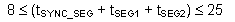Now, we calculate the reload and actual bit rate: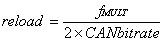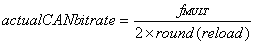All useful frequencies will have an error of less than 0.005.

#### Example of unusable crystal for 1Mbps CAN bit rate:

reload = 36.864E6 / (2*1E6) = 18.432

actual bit rate = 36.864E6 / (2*18) = 1024000

error = (1024000 - 1000000) / 1000000 = 0.024

#### Example of acceptable crystal for 1Mbps CAN bit rate:

reload = 36E6 / (2*1E6) = 18

actual bit rate = 36E6 / (2*18) = 1000000

error = (1000000 - 1000000) / 1000000 = 0.0

(tSEG1 + tSEG2 + tSYNC_SEG) = 18 / BPR

Remembering that tSYNC_SEG is always 1, and choosing 1 for BPR gives:

(tSEG1 + tSEG2) = 17

The designer picks the appropriate TSEG values from this constraint.

### Async Serial Using Timer 2

Bit rates for Serial Port 0 using Timer 2 are calculated while Timer 2 is in 16-bit autoreload mode. Timer 2 runs off the crystal inputs and is not affected by any crystal multiplier settings. For more detailed information, refer to Section 12 of the High-Speed Microcontroller User's Guide.

The bit rate is determined by the following equations: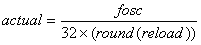All useful frequencies will have an error of less than 0.025.

#### Example of unusable crystal for 115200 bit/s:

reload = 16E6 / (32 x 115200) = 4.340

actual = 16E6 / (32 x 4) = 125000

error = (125000 - 115200) / 115200 = 0.0851

#### Example of acceptable crystal for 115200 bit/s:

reload = 18E6 / (32 x 115200) = 4.883

actual = 18E6 / (32 x 5) = 112500

error = (112500 - 115200) / 115200 = 0.0234

### Async Serial Using Timer 1

Bit rates for Serial Ports 0 and 1 using Timer 1 are calculated while Timer 1 is in 8-bit autoreload mode. An added feature of this process is the ability to generate baud rates based on divide-by-4 or divide-by-12 from the external crystal or the system clock. In divide-by-4 mode, the input clock is generated from the system clock (f MULT), while in divide-by-12, the base frequency of the external crystal will be used (f osc). You can also enable a serial baud-rate doubler by setting the appropriate SMOD (SMOD_0 or SMOD_1) bit. For more detailed information, refer to Section 12 of the High-Speed Microcontroller User's Guide.

#### Timer 1 in Divide-by-4 Mode

The bit rate is determined by the following equations: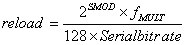All useful frequencies will have an error of less than 0.025

#### Example of unusable crystal:

reload = 20E6 / (64 x 115200) = 2.713

actual = 20E6 / (64 x 3) = 104167

error = (104167 - 115200) / 115200 = 0.096

#### Example of acceptable crystal:

reload = 22E6 / (64 x 115200) = 2.984

actual = 22E6 / (64 x 3) = 114583

error = (114583 - 115200) / 115200 = 0.0054

#### Timer 1 in Divide-by-12 Mode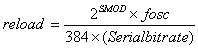All useful frequencies will have an error of less than 0.025

#### Example of unusable crystal for 115200 bit/s:

reload = 18E6 / (192 x 115200) = 0.814

actual = 18E6 / (192 x 1) = 93750

error = (93750 - 115200) / 115200 = 0.186

#### Example of acceptable crystal for 115200 bit/s:

reload = 22E6 / (192 x 115200) = 0.995

actual = 22E6 / (192 x 1) = 114583

error = (114583 - 115200) / 115200 = 0.0054

### Suggested Crystal Values for 115200 Serial and CAN Bus

The following table lists crystal frequencies that allow 115,200bps asynchronous serial using Serial 0 and Timer 2 and a 1Mb CAN bus.

Note: These values assume that the designer is not using the system clock multiplier.

 CRYSTAL CAN ERROR (%) SERIAL ERROR (%) 18000000 0.00 2.34 22000000 0.00 0.54 26000000 0.00 0.76 30000000 0.00 1.73 34000000 0.00 2.48 36000000 0.00 2.34 40000000 0.00 1.36 44000000 0.00 0.54 48000000 0.00 0.16 52000000 0.00 0.76 54000000 0.00 2.34 56000000 0.00 1.27 60000000 0.00 1.73 64000000 0.00 2.12 66000000 0.00 0.54 68000000 0.00 2.48 72000000 0.00 2.34